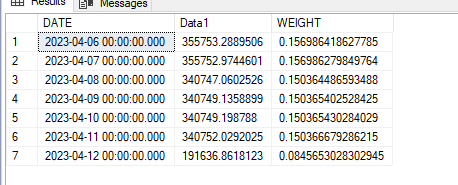# SQL query how to take out the average weight for the column

Hi Experts
Week starts from every wednesday to thursday sdql first reterive the daily data from wednesday to thrusday in one Data1 column then sum the values of Data1column and divided each daily value with sum of wed- Thruday value as shown in Weight column , after that multiple Weight column with Data2 column the final results are in weight data2 column.

hi

please provide the data in consumable format

create table
insert table

Hi Sir ,

``````IF OBJECT_ID('Table1') IS NOT NULL DROP TABLE [Table1]
GO
SELECT * INTO [Table1] FROM (SELECT CAST('06-Apr-2023 00:00:00' AS DATETIME) AS [Date], CAST('355753.2889506' AS FLOAT) AS [Data1], CAST('0.156986418627785' AS FLOAT) AS [Weight], CAST('0.165' AS FLOAT) AS [Data2], CAST('0.0259027590735845' AS FLOAT) AS [Weighted Data2] UNION ALL
SELECT CAST('07-Apr-2023 00:00:00' AS DATETIME) AS [Date], CAST('355752.9744601' AS FLOAT) AS [Data1], CAST('0.156986279849764' AS FLOAT) AS [Weight], CAST('0.187' AS FLOAT) AS [Data2], CAST('0.029356434331906' AS FLOAT) AS [Weighted Data2] UNION ALL
SELECT CAST('08-Apr-2023 00:00:00' AS DATETIME) AS [Date], CAST('340747.0602526' AS FLOAT) AS [Data1], CAST('0.150364486593488' AS FLOAT) AS [Weight], CAST('0.167' AS FLOAT) AS [Data2], CAST('0.0251108692611125' AS FLOAT) AS [Weighted Data2] UNION ALL
SELECT CAST('09-Apr-2023 00:00:00' AS DATETIME) AS [Date], CAST('340749.1358899' AS FLOAT) AS [Data1], CAST('0.150365402528425' AS FLOAT) AS [Weight], CAST('0.158' AS FLOAT) AS [Data2], CAST('0.0237577335994911' AS FLOAT) AS [Weighted Data2] UNION ALL
SELECT CAST('10-Apr-2023 00:00:00' AS DATETIME) AS [Date], CAST('340749.198788' AS FLOAT) AS [Data1], CAST('0.150365430284029' AS FLOAT) AS [Weight], CAST('0.16' AS FLOAT) AS [Data2], CAST('0.0240584688454446' AS FLOAT) AS [Weighted Data2] UNION ALL
SELECT CAST('11-Apr-2023 00:00:00' AS DATETIME) AS [Date], CAST('340752.0292025' AS FLOAT) AS [Data1], CAST('0.150366679286215' AS FLOAT) AS [Weight], CAST('0.185' AS FLOAT) AS [Data2], CAST('0.0278178356679497' AS FLOAT) AS [Weighted Data2] UNION ALL
SELECT CAST('12-Apr-2023 00:00:00' AS DATETIME) AS [Date], CAST('191636.8618123' AS FLOAT) AS [Data1], CAST('0.0845653028302945' AS FLOAT) AS [Weight], CAST('0.153' AS FLOAT) AS [Data2], CAST('0.0129384913330351' AS FLOAT) AS [Weighted Data2] UNION ALL
SELECT CAST(NULL AS DATETIME) AS [Date], CAST('2266140.549356' AS FLOAT) AS [Data1], CAST('1' AS FLOAT) AS [Weight], CAST('0.167857142857143' AS FLOAT) AS [Data2], CAST('0.168942592112523' AS FLOAT) AS [Weighted Data2] ) a

Select * from Table1
``````

hi

how does DATA2 , Weighted DATA2 columns get calculated ?

create sample data script

IF OBJECT_ID('Table1') IS NOT NULL DROP TABLE [Table1]
GO
SELECT * INTO [Table1] FROM
(
SELECT CAST('06-Apr-2023 00:00:00' AS DATETIME) AS [Date], CAST('355753.2889506' AS FLOAT) AS [Data1] UNION ALL
SELECT CAST('07-Apr-2023 00:00:00' AS DATETIME) AS [Date], CAST('355752.9744601' AS FLOAT) AS [Data1]UNION ALL
SELECT CAST('08-Apr-2023 00:00:00' AS DATETIME) AS [Date], CAST('340747.0602526' AS FLOAT) AS [Data1] UNION ALL
SELECT CAST('09-Apr-2023 00:00:00' AS DATETIME) AS [Date], CAST('340749.1358899' AS FLOAT) AS [Data1] UNION ALL
SELECT CAST('10-Apr-2023 00:00:00' AS DATETIME) AS [Date], CAST('340749.198788' AS FLOAT) AS [Data1] UNION ALL
SELECT CAST('11-Apr-2023 00:00:00' AS DATETIME) AS [Date], CAST('340752.0292025' AS FLOAT) AS [Data1] UNION ALL
SELECT CAST('12-Apr-2023 00:00:00' AS DATETIME) AS [Date], CAST('191636.8618123' AS FLOAT) AS [Data1]
) a

``````Select
[DATE]
, [Data1]
, [Data1]/sm as WEIGHT
from
Table1
, ( SELECT SUM(Data1) as sm from [Table1] ) a
``````Data1 column having input values and Data2 column having input values column Weight calculated as Sum of Data1 column divided by each values dates start from 6/6/2023 to 12/4/2023 .
Weighted Data2 column calculated as Weight * Data2.

This is weekly data the week starts from Wednesday to Thursday

Sir with respect ! is my previous post difficult to understand ok i accept my fault ...
but you are the senior member of this forum is this allow you to talk like that ?

If the senior members here talk like that please my aplogize delete my account from here , Every one here to do respect have respect.

See it i explained may be you missed out and having anger for no reason, Take a cup of coffee and review my highlighted area of post 5

ok

if you feel that way
good luck